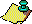Contents Next: Orbits under are Up: Continued Fractions and Chaos Previous: Ergodic Results

# The Floating-Point Gauss Map

All of the results of the previous sections are valid for the familiar domain of the real numbers. However, when we work in any fixed-precision system, we have two difficulties:
1. Not all numbers are even representable in the system, and
2. Arithmetic doesn't have the properties we are used to.
For example, defining u as the smallest machine representable number which when added to 1 gives a number different from 1 when stored, we see that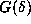is computed as 0, wheneveris any number between 0 and u. This effectively limits the power of the singularity of the Gauss map. Click here for further remarks on the machine epsilon u.

To return to the analogy of the introduction, we consider the domain of machine representable numbers not as a smooth circle but as a slotted ring, with the number of slots on the ring corresponding to the number of machine-representable numbers in the interval, where all numbers in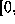uare ``lumped together''. In this analogy, u corresponds to the approximate width of the slots. Now our bead can occupy one of the slots on the ring, and not just any arbitrary position, and the floating-point Gauss map takes the bead from one slot, winding around the ring as many times as are indicated by the integer part, and finally putting the bead into another slot. We see now that the maximum winding number of the floating-point Gauss map is finite, and the slot next to the origin is the one with this winding number.

A more evident difficulty is that all the representable points are rational, and we know that the exact Gauss map takes these initial points to zero eventually. So if we define a floating-point Gauss map as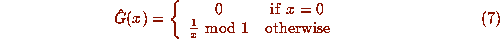where now the operations of division and ``mod 1'' take place over the floating-point domain, with attendant roundoff error, we have to answer some new dynamical questions.
1. Are there any orbits which don't go to 0?
2. Is the behaviour of the floating-point Gauss map similar to the exact Gauss map? In particular, is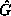chaotic?
3. Can we define an appropriate Lyapunov exponent for this map?
4. Is numerical work withuseful at all for study of G?

Not surprisingly, some orbits underdo terminate at 0, though often not when we expect them to. However, on some machines, some orbits never hit 0, being periodic. For example on the HP28S the initial point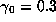gives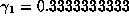,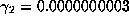, and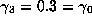, with period 3. Note that under the exact Gauss map the second iterate (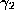) of this initial point is zero. Since the set of machine-representable numbers is finite, all orbits are ultimately periodic (perhaps with period 1, as at x=0). Note that the behaviour ofdepends strongly on the floating-point implementation. For example, with the Apple SANE numerics implementation, the starting pointgives an orbit with either a long transient regime or a long period, with no regularity detected in the first 65,000 elements of the orbit. A Maple implementation of Floyd's algorithm for finding when the cycle starts.

Since all orbits are ultimately periodic, and there are only a finite number of such orbits, the floating-point Gauss map (and indeed any machine simulation of any dynamical system) is not chaotic in the usual sense. Arbitrarily small perturbations in the initial conditions are not even allowed, so the sensitivity of the map to such perturbations is moot. The alternate definition of S. I. C. requires arbitrarily close initial conditions, which cannot happen in a finite set. Similarly, the usual definition of the Lyapunov exponent for the exact Gauss map seems not to be relevant here: the presence of the derivative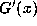in the definition of the Lyapunov exponent measures the effect of arbitrarily small perturbations. However, if we define an approximate Lyapunov exponent for the first N iterations of the floating point Gauss map as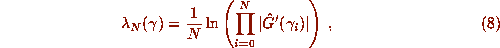whenever the elements of the orbit are nonzero, then this in some sense measures the average sensitivity of the first N elements of the corresponding orbit under the exact Gauss map to arbitrarily small perturbations. This ``Lyapunov exponent'' is what is calculated in practice for a great many numerical simulations of dynamical systems, and if it is positive this is taken as evidence for chaos in the underlying system .

But what if the calculated orbit has no counterpart in the exact system? If roundoff errors introduced into the calculation produce an orbit that is unlike any in the exact system, this approximate Lyapunov exponent would be spurious. We will give a proof in the following section, which uses the techniques of floating-point error analysis, that shows orbits under the floating-point Gauss map are ``machine close'' to corresponding orbits under the exact Gauss map. A general theorem of this nature has been proved for hyperbolic invariant sets, by Bowen (see ). Here a direct proof is more appropriate and informative. This means that the approximate Lyapunov exponent defined above will accurately reflect the Lyapunov exponent of some orbit of the exact Gauss map, provided N is large enough that transient effects have been minimized, and not so large that accumulated roundoff error in the sum degrades the result. This is usually not a large problem.

We contrast this behaviour with what happens when continuous maps are made discrete by (e.g.) finite difference schemes. Yamaguti & Ushiki  and Ushiki  have shown that finite difference formulae applied to non-chaotic continuous systems may produce chaotic numerical solutions if the stepsize is not too small, assuming that the calculations are carried out exactly. In contrast we have noted here that a chaotic discrete map becomes nonchaotic when implemented in fixed-precision arithmetic. A further difficulty is that all of the orbits ofare ultimately periodic, and periodic orbits of G have Lyapunov exponents that are different from the almost-everywhere value (which is usually the exponent of physical interest).

It is not immediately clear that these Lyapunov exponents calculated fromwill tell us anything useful about the exact map G.

On closer examination, however, we see that if the period of an orbit is long, then the orbit behaves for a long time as if it were aperiodic, reflecting the effect of `nearby' initial points that are aperiodic.

Hence we may expect that the computed Lyapunov exponent of a long period orbit will be close to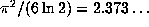. This is what happens in practice, since many initial points seem to give long period orbits. For example, if we compute the first 100,000 elements of the orbit of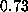underon the HP28S, we get a computed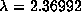. This is within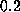% of the expected value of the Lyapunov exponent of the exact Gauss map. Notice, though, that the Lyapunov exponent of the orbit of the exact map G starting atis not even defined---we rely on the roundoff error to give us our results, which is somewhat unusual. We will expand more on this in a later section.Contents Next: Orbits under are Up: Continued Fractions and Chaos Previous: Ergodic Results